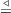#### Vol. 59, No. 2, 1975

 Download this articleFor screen For printingRecent Issues Vol. 325: 1 Vol. 324: 1  2 Vol. 323: 1  2 Vol. 322: 1  2 Vol. 321: 1  2 Vol. 320: 1  2 Vol. 319: 1  2 Vol. 318: 1  2Online Archive Volume: Issue:The Journal Subscriptions Editorial Board Officers Contacts Submission Guidelines Submission Form Policies for Authors ISSN: 1945-5844 (e-only) ISSN: 0030-8730 (print) Special Issues Author Index To Appear Other MSP Journals
A characterization of the symplectic groups PSp(2m, q) as rank 3 permutation groups

### Arthur Anthony Yanushka

Vol. 59 (1975), No. 2, 611–621
##### Abstract

In this paper the following characterization of the symplectic groups PSp(2m,q) for m > 2 as rank 3 permutation groups is obtained:

THEOREM. Let G be a transitive rank 3 group of permutations of a finite set X such that the orbit lengths for Gb, the stabilizer of a point b in X are 1, q(qr2 1)(q 1) and qr1 for integers q > 1 and r > 4. Let b denote the union of b and the Gb orbit of length q(qr2 1)(q 1). Let R(bc) denote ∩{z : b,c z}. Assume R(bc){b,c}, for all distinct pairs of points, b and c. Assume that the pointwise stabilizer of b is transitive on the points unequal to b of R(bc) for cb. Then r is even, q is a prime power and GH, a group of symplectic collineations of projective r 1 space over the finite field of q elements and PSp(r,q)H.

Primary: 20B10
##### Milestones